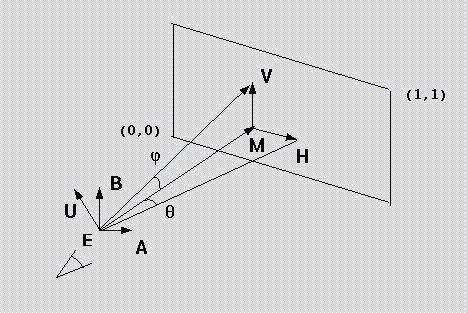# A Simple Viewing Geometry

From Graphics Gems II, James Arvo, ed. Academic Press, Boston, 1991.
3D Geometry and Algorithms Section
Gem IV.4
Andrew S. Glassner
Xerox PARC
Palo Alto, California
pp 179-180

Sometimes, it is handy to be able to set up a simple viewing geometry. For example, packages without matrix libraries, quick preview hacks, and simple ray tracers all can benefit from a simple viewing construction. Here is one that I have found handy.

The input is a viewpoint E, a gaze direction and distance G, and up vector U, and viewing half-angles theta and phi. The output is a screen midpoint M and two vectors, H and V, which are used to sweep the screen. Figure 1 shows the setup.

First, create vector A by A = G x U. (I assume right-handed cross products.) Then find B from B = A x G. Vector B is coplanar with U and G, but it is orthogonal to A and G. The midpoint of the screen is found from M = E += G. (Note that the length of G tells you how far away the viewscreen is located.) Vectors A and B span the viewplane, but they are the wrong size. Find the vector H by scaling A by the horizontal half-angle: H = ( A |G| tan(phi)) / |A|. Similarly, the vertical vector is found by V = ( B |G| tan(theta))/|B|.

Assuming your origin is in the lower left, as shown, then any point S on the screen may be specified by (sx, sy), both numbers between 0 and 1. (For example, if your frame buffer is 640 pixels wide y 480 pixels high, then (30, 250) would map to 30/639, 250/479)). The point P on the image plane then is P = M + (2*sx - 1)*H + (2*sy - 1)*V. If you oprefer to have your origin in the upper left, then generate points with the equation P = M + (2*sx - 1)*H + (1 - 2*sy)*V.

If you are ray tracing, the ray equation would be R = E + (P - E)*t. (You may with to normalize (P-E) before actually shooting the ray.)The following table compares the parameters given in the header file scene_io.h (and a few other quantities) with Glassner's geometry described above:

scene_io.h Glassner description
position E viewpoint
viewDirection G/|G| gaze direction
orthoUp B/|B| up, orthogonal to gaze direction
focalDistance |G| distance to focal plane
verticalFOV 2*phi vertical perspective angle
(aspectRatio) |H|/|V| aspect ratio (always 1 for HW#1)

Tamara Munzner